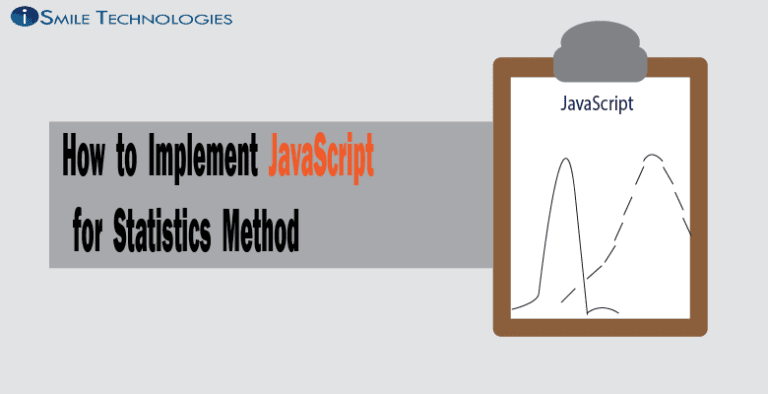# How to Implement JavaScript for Statistics Method

Over these past few weeks, I’ve learned that JavaScript is an extremely useful programming language. In this blog, we will see how we can use JavaScript to implement statistical methods into our programs.As you can probably imagine, there exist a couple libraries for statistical methods inside JavaScript. One of them is Simple Statistics. Here’s the link for the documentation for this library:
https://simplestatistics.org/

I will give a basic demonstration of some of the simpler statistical methods.

## min and max

```				```
min(x: Array<number>): number
```
```

(max is written in the same way, just swap min with max)

min and max return the smallest/largest number in an array. The only parameter is that you input an array of numbers.

## sum

```				```
sum(x:Array<number>): number
```
```

sum returns the sum of all the numbers in an array. Again, the only parameter is that you input an array of numbers.

## mean

```				```
mean(x: Array<number>): number
```
```

Mean returns the average of all the numbers in an array. The only parameter is that you input an array of numbers.

## variance

```				```
variance(x: Array<number>): number
```
```

Variance returns the sum of squared deviations from all the numbers in an array. The only parameter is that you input an array of numbers.

## zScore

```				```
zScore(x: number, mean: number, standardDeviation: number): number
```
```

zScore measures the score above or below the mean. For this, you need only provide the x (observed value), the mean (mu), and the standard deviation (sigma).

As you can see from these examples, Simple Statistics is a very intuitive JavaScript library. If you have any interest in this library, you can install it with this terminal command (assuming you have node.js):

```				```
npm install --save simple-statistics
```
```

Again, here is the link to the documentation if you need it:
https://simplestatistics.org/

In researching for this blog, I also found another library, jstat. This blog is already long enough, so I will not be delving into it, but here’s the documentation for that library if you need it:
https://jstat.github.io/overview.html

This has been Warren Ball, data science intern at iSmile Technologies. Thanks for reading, I hope this helps.### Get free consultation from our tech experts## Related articles you may would like to read

##### Will Driving Automation Become a Vital Part of The Future?##### Autonomous Car in Automotive### FREE eBOOK: Recommended RFP process for selecting cloud provider

We are here to simply your migration to cloud by embarking on a systematic, planned, stage-wise journey to the cloud.

##### Categories